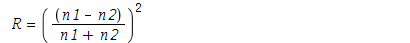4 years, 39 days

## How to plot complex equation |z+1||z-1|=...

Maple 2020

How do I plot the complex function |z+1||z-1|=1 in Maple? I know there is complexplot but don't quite understand how to use it.

## Unable to create Group from a cayley tab...

Maple 2019

Hi, I am using the GroupTheory package and I wanted to created the following group in Maple:I stumbled across this link https://www.maplesoft.com/products/maple/features/grouptheory.aspx and then tried to use the following commands to define this group in Maple:

1. First a defined a 12x12 matrix:

ct := <<e | p | q | r | s | t | u | v | w | x | y | z>, <p | q | e | y | u | w | z | r | x | t | v | s>, <q | e | p | v | z | x | s | y | t | w | r | u>, <r | z | t | s | e | y | v | x | p | u | q | w>, <s | w | y | e | r | q | x | u | z | v | t | p>, <t | r | z | x | w | u | e | q | y | p | s | v>, <u | x | v | p | y | e | t | z | s | r | w | q>, <v | u | x | z | q | r | y | w | e | s | p | t>, <w | y | s | t | x | z | p | e | v | q | u | r>, <x | v | u | w | t | s | q | p | r | e | z | y>, <y | s | w | u | p | v | r | t | q | z | e | x>, <z | t | r | q | v | p | w | s | u | y | x | e>>

2. Then I tried to define my  group using:

G := Group(ct)

However this doesn't work because I get the following error:

`Error, (in GroupTheory:-Group) invalid input: arguments to GroupTheory:-Group, [Matrix(12, 12, {(1, 1) = e, (1, 2) = p, (1, 3) = q, (1, 4) = r, (1, 5) = s, (1, 6) = t, (1, 7) = u, (1, 8) = v, (1, 9) = w, (1, 10) = x, (1, 11) = y, (1, 12) = z, (2, 1) = p, (2, 2) = q, (2, 3) = e, (2, 4) = y, (2, 5) = u, (2, 6) = w, (2, 7) = z, (2, 8) = r, (2, 9) = x, (2, 10) = t, (2, 11) = v, (2, 12) = s, (3, 1) = q, (3, 2) = e, (3, 3) = p, (3, 4) = v, (3, 5) = z, (3, 6) = x, (3, 7) = s, (3, 8) = y, (3, 9) = t, (3, 10) = w, (3, 11) = r, (3, 12) = u, (4, 1) = r, (4, 2) = z, (4, 3) = t, (4, 4) = s, (4, 5) = e, (4, 6) = y, (4, 7) = v, (4, 8) = x, (4, 9) = p, (4, 10) = ...  (12, 8) = s, (12, 9) = u, (12, 10) = y, (12, 11) = x, (12, 12) = e})], do not match any of the accepted calling sequences`

## How to create a group with known element...

Maple 2019

I would like to know how to create a group with some elements (numbers) {1,2,4,...} with a defined operation on it like X_42 (modular  multiplication by 42).

## How to evaluate this type of contour int...

Maple 2018

So I have got the following integral:around the square with corners 2, 2i, -2, -2i oriented counter-clockwise.

Do I need to tell Maple that z is complex? Do I need to manually parametrize z? Is Maple aware of Cauchy's theorem?

What is the quickest way of evaluating this sort of integrals.

## Plot function as a ratio of two variable...

Maple 2018

I have got the following function:

R = ((n1-n2)/(n1+n2))^2I would like to plot R as a function of (n1/n2). How can I do this in maple?1 2 Page 2 of 2
﻿